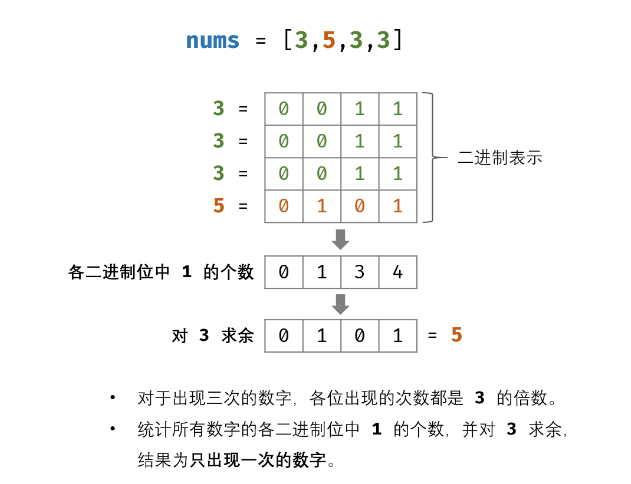## 思路：O(k)

## 题解：

``````class Solution {
public:
int singleNumber(vector<int>& nums) {
int count;
memset(count,0,sizeof(count));
for(int it:nums){
for(int i=0;i<32;i++){//统计数字在各32位中1的数量
count[i]+=(1&it);
it>>=1;
}
}
int res=0;
for(int i=0;i<32;i++){
res+=(1<<i)*(count[i]%3);//实际上 根据题设的不同，3可以任意更改
}
return res;
}
};``````